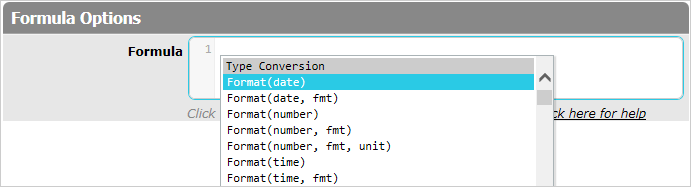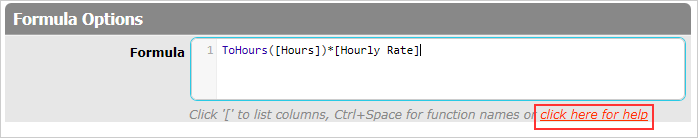# Functions

Functions, similarly to operators, represent certain predetermined operations that will be carried out when formula result is calculated. Functions consist of a function name and a list of function arguments (separated by commas) in parentheses. Function arguments are values that will be used to calculate the function result; for arguments you may use literals, column references or variables. Press `Ctrl + Space` to list function names and select the functions you need.Functions, like column references, may be entered manually; however, unlike references, they are case sensitive.

For example, the `Sum` function is used to sum up several values listed in parentheses. For its two arguments you can use literals, column references or variables. The `Sum([Salary], 300)` function, where `[Salary]` and `300` act as arguments, will add a bonus of 300 to the value retrieved from the "Salary" column.

If needed, functions can be nested within each other.

If you need information about the Formula Language Reference, use the `click here for help` link:Use these functions when building formulas.